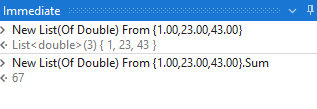# Sum of list values

How can I return sum of List values that contains doubles?

1 Like

Hi @oliveria,

If the List is of type Double, then this ought to do the trick →``````lst_values.Sum
``````

If that doesn’t do the trick, then please provide us with some sample data so that we can test it out from out end.

Kind Regards,
Ashwin A.K

Try this expression

``````(From d in dtData.AsEnumerable Where Not (isNothing(d(ColumnNameorIndex)) OrElse String.IsNullorEmpty(d(ColumnNameorIndex).toString.Trim)) Select v = CDbl(d(ColumnNameorIndex).toString.Trim)).Sum(Function (x) x)
``````

Regards
Gokul

Hi

Let’s take a simple expression

Doub_output = ListVariable.Sum(function(x) Convert.ToDouble(x) )

Cheers @Olivera_Kalinic

It works1 Like